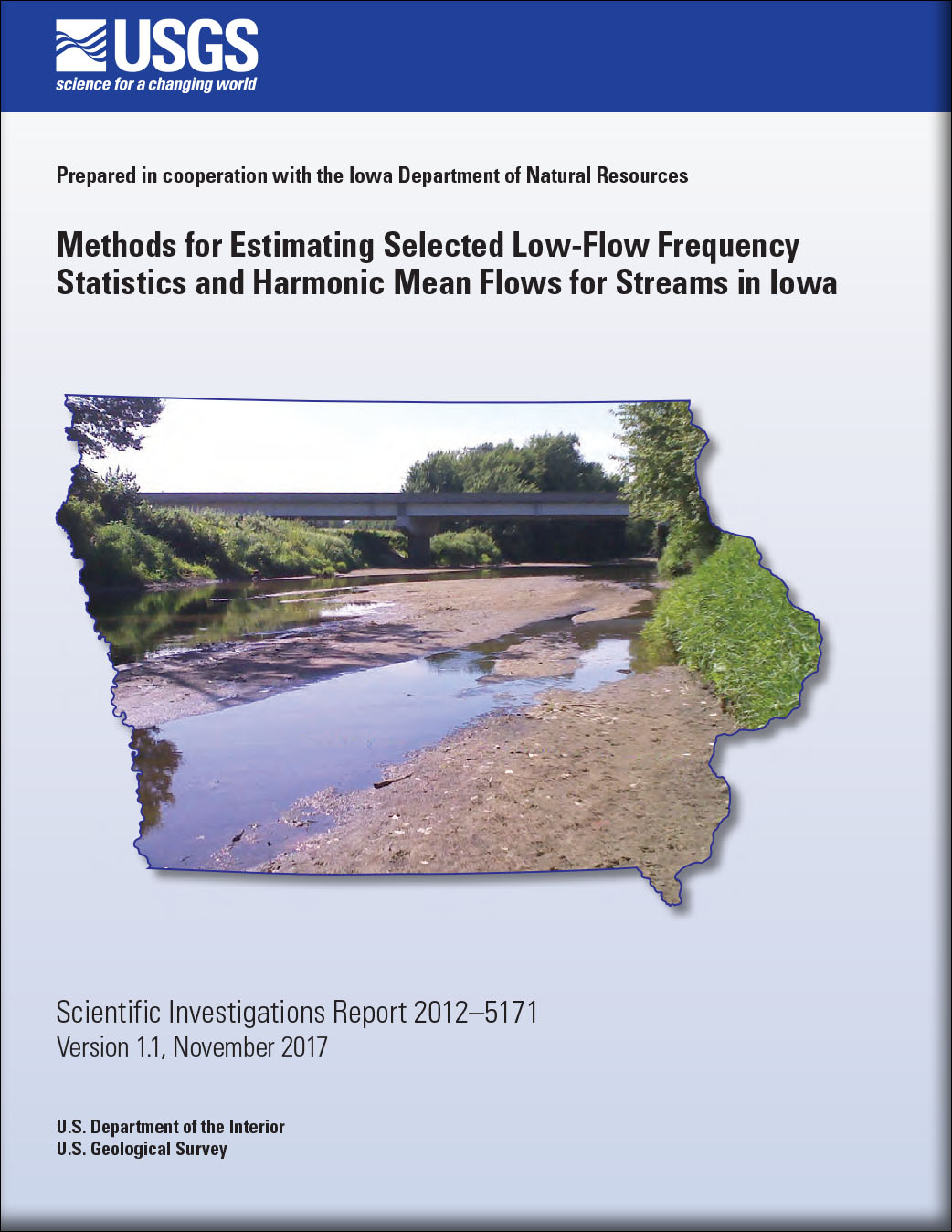Scientific Investigations Report 2012–5171

## Methods for Estimating Selected Low-Flow Frequency Statistics and Harmonic Mean Flows for Streams in Iowa

###Abstract

A statewide study was conducted to develop regression equations for estimating six selected low-flow frequency statistics and harmonic mean flows for ungaged stream sites in Iowa. The estimation equations developed for the six low-flow frequency statistics include: the annual 1-, 7-, and 30-day mean low flows for a recurrence interval of 10 years, the annual 30-day mean low flow for a recurrence interval of 5 years, and the seasonal (October 1 through December 31) 1- and 7-day mean low flows for a recurrence interval of 10 years. Estimation equations also were developed for the harmonic-mean-flow statistic. Estimates of these seven selected statistics are provided for 208 U.S. Geological Survey continuous-record streamgages using data through September 30, 2006. The study area comprises streamgages located within Iowa and 50 miles beyond the State’s borders. Because trend analyses indicated statistically significant positive trends when considering the entire period of record for the majority of the streamgages, the longest, most recent period of record without a significant trend was determined for each streamgage for use in the study. The median number of years of record used to compute each of these seven selected statistics was 35. Geographic information system software was used to measure 54 selected basin characteristics for each streamgage. Following the removal of two streamgages from the initial data set, data collected for 206 streamgages were compiled to investigate three approaches for regionalization of the seven selected statistics. Regionalization, a process using statistical regression analysis, provides a relation for efficiently transferring information from a group of streamgages in a region to ungaged sites in the region. The three regionalization approaches tested included statewide, regional, and region-of-influence regressions. For the regional regression, the study area was divided into three low-flow regions on the basis of hydrologic characteristics, landform regions, and soil regions. A comparison of root mean square errors and average standard errors of prediction for the statewide, regional, and region-of-influence regressions determined that the regional regression provided the best estimates of the seven selected statistics at ungaged sites in Iowa.

Because a significant number of streams in Iowa reach zero flow as their minimum flow during low-flow years, four different types of regression analyses were used: left-censored, logistic, generalized-least-squares, and weighted-least-squares regression. A total of 192 streamgages were included in the development of 27 regression equations for the three low-flow regions. For the northeast and northwest regions, a censoring threshold was used to develop 12 left-censored regression equations to estimate the 6 low-flow frequency statistics for each region. For the southern region a total of 12 regression equations were developed; 6 logistic regression equations were developed to estimate the probability of zero flow for the 6 low-flow frequency statistics and 6 generalized least-squares regression equations were developed to estimate the 6 low-flow frequency statistics, if nonzero flow is estimated first by use of the logistic equations. A weighted-least-squares regression equation was developed for each region to estimate the harmonic-mean-flow statistic. Average standard errors of estimate for the left-censored equations for the northeast region range from 64.7 to 88.1 percent and for the northwest region range from 85.8 to 111.8 percent. Misclassification percentages for the logistic equations for the southern region range from 5.6 to 14.0 percent. Average standard errors of prediction for generalized least-squares equations for the southern region range from 71.7 to 98.9 percent and pseudo coefficients of determination for the generalized-least-squares equations range from 87.7 to 91.8 percent. Average standard errors of prediction for weighted-least-squares equations developed for estimating the harmonic-mean-flow statistic for each of the three regions range from 66.4 to 80.4 percent.

The regression equations are applicable only to stream sites in Iowa with low flows not significantly affected by regulation, diversion, or urbanization and with basin characteristics within the range of those used to develop the equations. If the equations are used at ungaged sites on regulated streams, or on streams affected by water-supply and agricultural withdrawals, then the estimates will need to be adjusted by the amount of regulation or withdrawal to estimate the actual flow conditions if that is of interest. Caution is advised when applying the equations for basins with characteristics near the applicable limits of the equations and for basins located in karst topography. A test of two drainage-area ratio methods using 31 pairs of streamgages, for the annual 7-day mean low-flow statistic for a recurrence interval of 10 years, indicates a weighted drainage-area ratio method provides better estimates than regional regression equations for an ungaged site on a gaged stream in Iowa when the drainage-area ratio is between 0.5 and 1.4.

These regression equations will be implemented within the U.S. Geological Survey StreamStats web-based geographic-information-system tool. StreamStats allows users to click on any ungaged site on a river and compute estimates of the seven selected statistics; in addition, 90-percent prediction intervals and the measured basin characteristics for the ungaged sites also are provided. StreamStats also allows users to click on any streamgage in Iowa and estimates computed for these seven selected statistics are provided for the streamgage.

First posted August 27, 2012

Director, Iowa Water Science Center
U.S. Geological Survey
P.O. Box 1230
Iowa City, IA 52244
http://ia.water.usgs.gov

### Suggested citation:

Eash, D.A., and Barnes, K.K., 2017, Methods for estimating selected low-flow frequency statistics and harmonic mean flows for streams in Iowa (ver 1.1, November 2017): U.S. Geological Survey Scientific Investigations Report 2012–5171, 99 p., https://doi.org/10.3133/sir20125171.

### Contents

Abstract

Introduction

Methods for Data-Set Development for Streamgages

Regional Regression Analyses to Estimate Selected Low-Flow Frequency Statistics and Harmonic Mean Flows for Ungaged Stream Sites

Region-of-Influence Method to Estimate Selected Low-Flow Frequency Statistics and Harmonic Mean Flows for Ungaged Stream Sites

Weighted Drainage-Area Ratio Method to Estimate Selected Low-Flow Frequency Statistics and Harmonic Mean Flows for Ungaged Sites on Gaged Streams

StreamStats

Summary

Acknowledgments

References Cited

Appendix 1U.S. Department of the Interior | U.S. Geological Survey
URL: https://pubs.usgs.gov/sir/2012/5171/